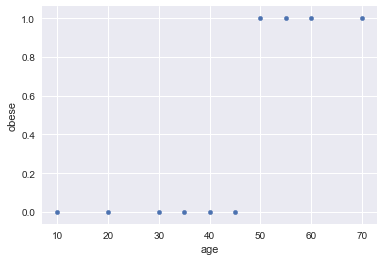# Interpreting Correlations¶

This is a learning exercise that teaches you how to analyze correlations within mixed data sets. In this exercise we will study the following:

1. Types of variables as they relate to statistics.
2. What correlation metrics are common.
3. Interpretation of correlation metrics.

## Variable Types¶

### 1. Numerical¶

Numerical values fall within two categories:

1. Discrete - the values are confined to a certain limit and are integers. An example could be a binary value consisting of 0/1.
2. Continuous - these values can be divided in a way to construct different scales to look at subpopulations. For example, a measurement of age within a population provides continuous values. When you are looking into the subpopulations within that data set, you can simply create custom ranges (ages 1 through 10) to look at distributions.

Here is some code to look at how these values look given their distribution:

In :
%matplotlib inline

import numpy as np
import seaborn as sns
import pandas as pd

In :
N = 1000

# Generate N random binary values
random_binary = np.random.choice([0, 1], size=(N,))

# Generate N random ages from 0 to 100
random_ages = np.random.randint(0, 100, N)

In :
# Plot binary distribution
ax = sns.distplot(random_binary)
ax.set_title('Binary Distribution')
print('mean', np.mean(random_binary))

mean 0.514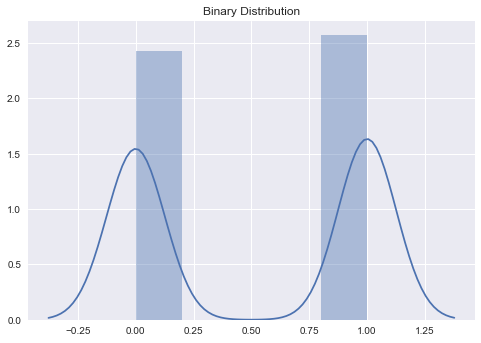The binary distribution has a mean of around 50%. As you provide more samples, you will see it converge closer to the mean (see Central Limit Thereom). The samples consist of an almost equal distribution of 0's and 1's for values.

In :
# Plot age distribution
ax = sns.distplot(random_ages)
ax.set_title('Continuous Distribution\nAges 0 to 100')
print('mean', np.mean(random_ages))

mean 50.326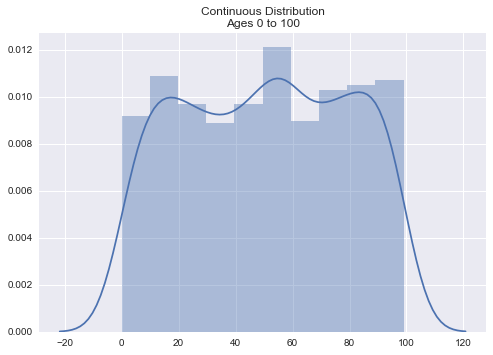Due to the range of values that we chose, the mean will converge at around 50. However, you can see that the distribution looks a much different than the binary distribution. This is due to the variability in the values.

### 2. Categorical¶

These types of numbers are simply used to group specific samples. They do not provide any mathematical meaning. For example, a 1 for male and 2 for female does not provide any importance.

### 3. Ordinal¶

Categorical values that provide weight with their meanings. It is essentially a mix of numerical and categorical values. For example, a ranking system.

For a race you can think of the following as individuals are given prizes:

• 1st place
• 2nd place
• 3rd place

or

A pain chart provided at a hospital in which 1 through 10 expresses the amount of pain you are experiencing.

## Common Correlation Metrics¶

### 1. Pearson¶

The Pearson Correlation Coeffecient measures the relationship of variables in a linear fashion on an X and Y coordinate system. The values range from -1 to +1. When the value is closest to -1, the relationship is negatively correlated. When the value is closest to +1, the relationship is positively correlated. Having a value close to 0 means that there is no significant correlation between X and Y.

Below we look at comparing age to salary with values that create a strong positive correlation.

In :
ages = [18, 18, 25, 27, 30, 38]
wages = [18000, 24000, 55000, 61000, 65000, 88000]

df = pd.DataFrame({'ages': ages, 'wages': wages})

In :
sns.lmplot('ages', 'wages', data=df)

Out:
<seaborn.axisgrid.FacetGrid at 0x7f5a9a7a25c0>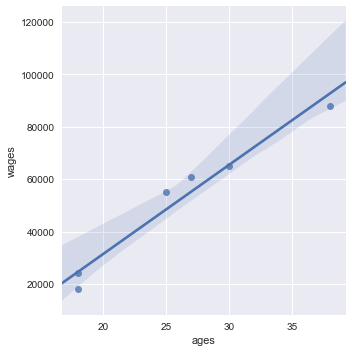In :
df.corr()

Out:
ages wages
ages 1.00000 0.97941
wages 0.97941 1.00000

You can see that these variables have a strong positive correlation. They are very close to 1.

### 2. Spearman¶

The Spearman Rank Order Correlation measures the relationship of ordinal, interval or ratio variables. These values must be monotonic in nature. Essentially the variables both decrease or increase together.

In :
x = np.arange(0, 100)
x2 = np.arange(1, 5)
y = (1/4) * (x**2)
y2 = np.exp(-x2)
y3 = np.sin(x / 5)

In :
# Plot increasing relation

df = pd.DataFrame({'x': x, 'y': y})
ax = sns.lmplot('x', 'y', data=df)
sns.plt.title('Monotonically Increasing')

Out:
<matplotlib.text.Text at 0x7f5a99673358>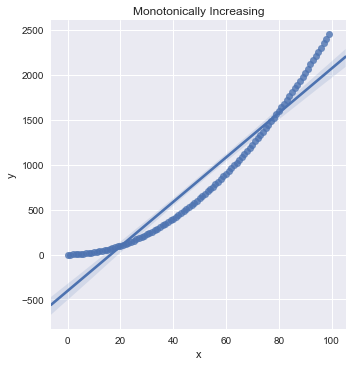In :
df.corr(method='spearman')

Out:
x y
x 1.0 1.0
y 1.0 1.0
In :
# Plot decreasing relation

df = pd.DataFrame({'x': x2, 'y': y2})
ax = sns.lmplot('x', 'y', data=df)
sns.plt.title('Monotonically Decreasing')

Out:
<matplotlib.text.Text at 0x7f5a995e65c0>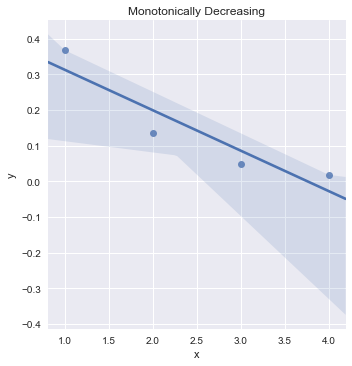In :
df.corr(method='spearman')

Out:
x y
x 1.0 -1.0
y -1.0 1.0
In :
# Plot no relation

df = pd.DataFrame({'x': x, 'y': y3})
ax = sns.lmplot('x', 'y', data=df)
sns.plt.title('Not Monotonic')

Out:
<matplotlib.text.Text at 0x7f5a9942aac8>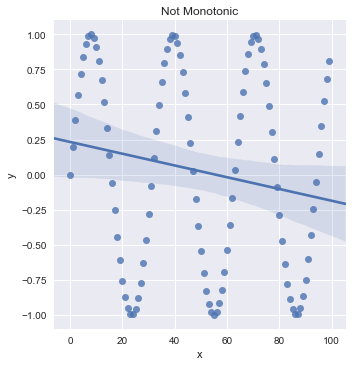In :
df.corr(method='spearman')

Out:
x y
x 1.000000 -0.172841
y -0.172841 1.000000

### 3. Biserial¶

A Point-biserial correlation consists of a binary variable and a non-binary variable. It is the same computation as the Pearson correlation with the exception of having a binary variable.

Let's create a distribution where you become obese as you get older.

In :
# Create some values
obese = [0, 0, 0, 0, 0, 0, 1, 1, 1, 1]
ages = [10, 20, 30, 35, 40, 45, 50, 55, 60, 70]

df = pd.DataFrame({'obese': obese, 'age': ages})

In :
df.corr()

Out:
age obese
age 1.00000 0.80615
obese 0.80615 1.00000
In :
df.plot.scatter('age', 'obese')

Out:
<matplotlib.axes._subplots.AxesSubplot at 0x7f5a993ee6a0>